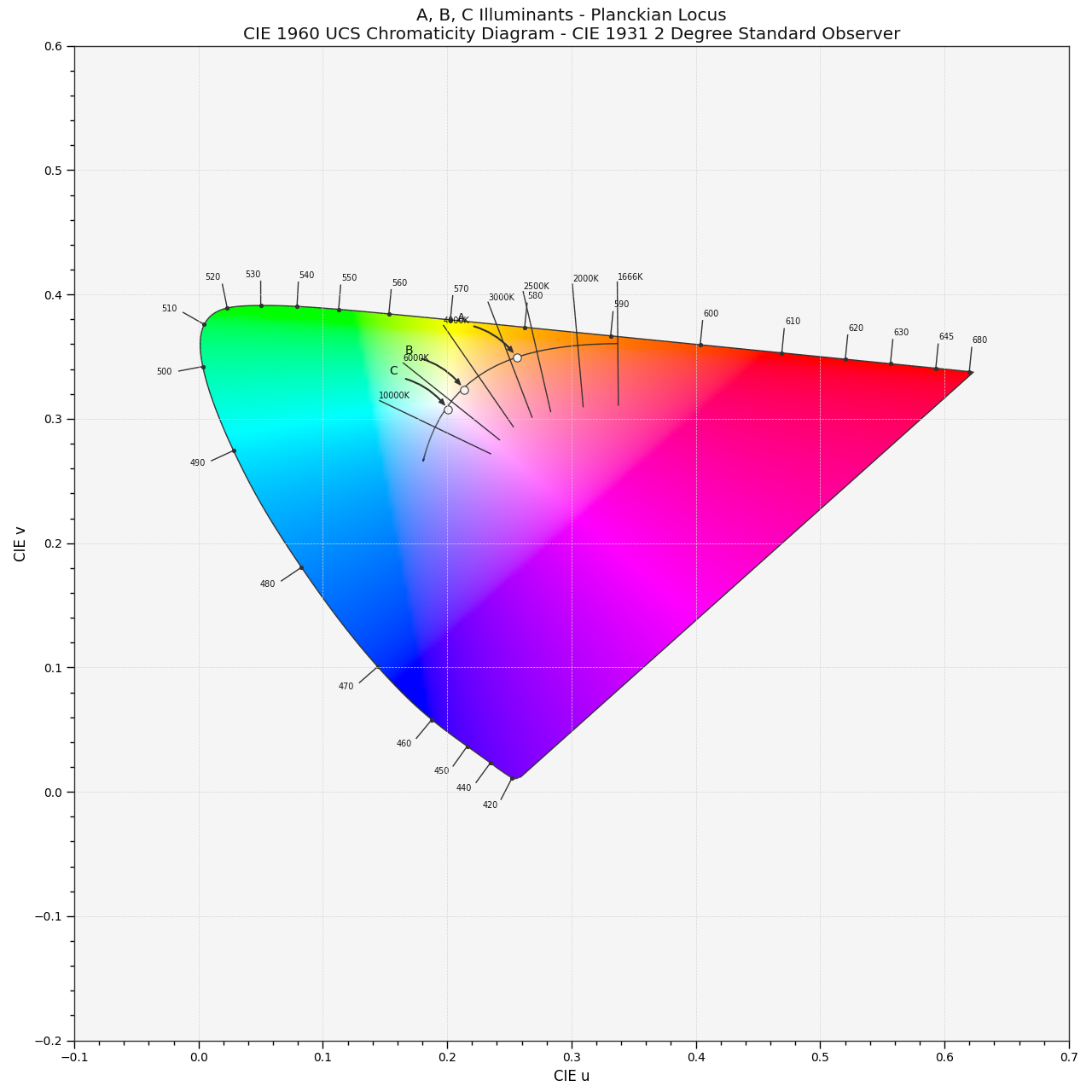# colour.plotting.plot_planckian_locus_in_chromaticity_diagram_CIE1960UCS¶

colour.plotting.plot_planckian_locus_in_chromaticity_diagram_CIE1960UCS(illuminants: Union[str, Sequence[str]], chromaticity_diagram_callable_CIE1960UCS: Callable = plot_chromaticity_diagram_CIE1960UCS, annotate_kwargs: Optional[Union[Dict, List[Dict]]] = None, plot_kwargs: Optional[Union[Dict, List[Dict]]] = None, **kwargs: Any) [source]

Plot the Planckian Locus and given illuminants in CIE 1960 UCS Chromaticity Diagram.

Parameters
Returns

Current figure and axes.

Return type

`tuple`

Examples

```>>> plot_planckian_locus_in_chromaticity_diagram_CIE1960UCS(
...     ['A', 'C', 'E'])
(<Figure size ... with 1 Axes>, <...AxesSubplot...>)
```